music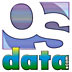# Assembly Language

## summary

This web page examines table instructions in assembly language. Specific examples of instructions from various processors are used to illustrate the general nature of assembly language.

## free computer programming text book project

If you like the idea of this project,Now building a For those with high speed connections, the very large single file summary is still on line.

### table operations

• TBLS Table Lookup and Interpolate (Signed, Rounded); Motorola 68300; signed lookup and interpolation of independent variable X from a compressed linear data table or between two register-based table entries of linear representations of dependent variable Y as a function of X; ENTRY(n) + {(ENTRY(n+1) - ENTRY(n)) * Dx[7:0]} / 256 into Dx; table version: data register low word contains the independent variable X, 8-bit integer part and 8-bit fractional part with assumed radix point located between bits 7 and 8, source effective address points to beginning of table in memory, integer part scaled to data size (byte, word, or longword) and used as offset from beginning of table, selected table entry (a linear representation of dependent variable Y) is subtracted from the next consecutive table entry, then multiplied by the interpolation fraction, then divided by 256, then added to the first table entry, and then stored in the data register; register version: data register low byte contains the independent variable X 8-bit fractional part with assumed radix point located between bits 7 and 8, two data registers contain the byte, word, or longword table entries (a linear representation of dependent variable Y), first data register-based table entry is subtracted from the second data register-based table entry, then multiplied by the interpolation fraction, then divided by 256, then added to the first table entry, and then stored in the destination (X) data register, the register interpolation mode may be used with several table lookup and interpolations to model multidimentional functions; rounding is selected by the ‘R’ instruction field, for a rounding adjustment of -1, 0, or +1; the result is an 8-, 16-, or 24-bit integer and eight-bit fraction; interpolation resolution is limited to 1/256th the distance between consecutrive table entries, X should be considered an integer in the range 0X65535; sets or clears flags
• TBLSN Table Lookup and Interpolate (Signed, Not Rounded); Motorola 68300; signed lookup and interpolation of independent variable X from a compressed linear data table or between two register-based table entries of linear representations of dependent variable Y as a function of X; ENTRY(n) * 256 + (ENTRY(n+1) - ENTRY(n)) * Dx[7:0] into Dx; table version: data register low word contains the independent variable X, 8-bit integer part and 8-bit fractional part with assumed radix point located between bits 7 and 8, source effective address points to beginning of table in memory, integer part scaled to data size (byte, word, or longword) and used as offset from beginning of table, selected table entry (a linear representation of dependent variable Y) multiplied by 256, then added to the value determined by (selected table entry subtracted from the next consecutive table entry, then multiplied by the interpolation fraction), and then stored in the data register; register version: data register low byte contains the independent variable X 8-bit fractional part with assumed radix point located between bits 7 and 8, two data registers contain the byte, word, or longword table entries (a linear representation of dependent variable Y), first data register-based table entry is multiplied by 256, then added to the value determined by (first data register-based table entry subtracted from the second data register-based table entry, then multiplied by the interpolation fraction), and then stored in the destination (X) data register, the register interpolation mode may be used with several table lookup and interpolations to model multidimentional functions; the result is an 8-, 16-, or 24-bit integer and eight-bit fraction; interpolation resolution is limited to 1/256th the distance between consecutrive table entries, X should be considered an integer in the range 0X65535; sets or clears flags
• TBLU Table Lookup and Interpolate (Unsigned, Rounded); Motorola 68300; unsigned lookup and interpolation of independent variable X from a compressed linear data table or between two register-based table entries of linear representations of dependent variable Y as a function of X; ENTRY(n) + {(ENTRY(n+1) - ENTRY(n)) * Dx[7:0]} / 256 into Dx; table version: data register low word contains the independent variable X, 8-bit integer part and 8-bit fractional part with assumed radix point located between bits 7 and 8, source effective address points to beginning of table in memory, integer part scaled to data size (byte, word, or longword) and used as offset from beginning of table, selected table entry (a linear representation of dependent variable Y) is subtracted from the next consecutive table entry, then multiplied by the interpolation fraction, then divided by 256, then added to the first table entry, and then stored in the data register; register version: data register low byte contains the independent variable X 8-bit fractional part with assumed radix point located between bits 7 and 8, two data registers contain the byte, word, or longword table entries (a linear representation of dependent variable Y), first data register-based table entry is subtracted from the second data register-based table entry, then multiplied by the interpolation fraction, then divided by 256, then added to the first table entry, and then stored in the destination (X) data register, the register interpolation mode may be used with several table lookup and interpolations to model multidimentional functions; rounding is selected by the ‘R’ instruction field, for a rounding adjustment of 0 or +1; the result is an 8-, 16-, or 24-bit integer and eight-bit fraction; interpolation resolution is limited to 1/256th the distance between consecutrive table entries, X should be considered an integer in the range 0X65535; sets or clears flags
• TBLUN Table Lookup and Interpolate (Unsigned, Not Rounded); Motorola 68300; unsigned lookup and interpolation of independent variable X from a compressed linear data table or between two register-based table entries of linear representations of dependent variable Y as a function of X; ENTRY(n) * 256 + (ENTRY(n+1) - ENTRY(n)) * Dx[7:0] into Dx; table version: data register low word contains the independent variable X, 8-bit integer part and 8-bit fractional part with assumed radix point located between bits 7 and 8, source effective address points to beginning of table in memory, integer part scaled to data size (byte, word, or longword) and used as offset from beginning of table, selected table entry (a linear representation of dependent variable Y) multiplied by 256, then added to the value determined by (selected table entry subtracted from the next consecutive table entry, then multiplied by the interpolation fraction), and then stored in the data register; register version: data register low byte contains the independent variable X 8-bit fractional part with assumed radix point located between bits 7 and 8, two data registers contain the byte, word, or longword table entries (a linear representation of dependent variable Y), first data register-based table entry is multiplied by 256, then added to the value determined by (first data register-based table entry subtracted from the second data register-based table entry, then multiplied by the interpolation fraction), and then stored in the destination (X) data register, the register interpolation mode may be used with several table lookup and interpolations to model multidimentional functions; the result is an 8-, 16-, or 24-bit integer and eight-bit fraction; interpolation resolution is limited to 1/256th the distance between consecutrive table entries, X should be considered an integer in the range 0X65535; sets or clears flags

Now building a For those with high speed connections, the very large single file summary is still on line.

# free music player coding example

Programming example: I am making heavily documented and explained open source PHP/MySQL code for a method to play music for free — almost any song, no subscription fees, no download costs, no advertisements, all completely legal. This is done by building a front-end to YouTube (which checks the copyright permissions for you).

View music player in action: www.musicinpublic.com/.

Create your own copy from the original source code/ (presented for learning programming). Includes how to run this from your own computer if you don’t have a web site.

### OSdata.com is used in more than 300 colleges and universities around the world

Some or all of the material on this web page appears in the

## view text bookHTML file

Because I no longer have the computer and software to make PDFs, the book is available as an HTML file, which you can convert into a PDF.

 Tweets by @osdata

A web site on dozens of operating systems simply can’t be maintained by one person. This is a cooperative effort. If you spot an error in fact, grammar, syntax, or spelling, or a broken link, or have additional information, commentary, or constructive criticism, please e-mail Milo. If you have any extra copies of docs, manuals, or other materials that can assist in accuracy and completeness, please send them to Milo, PO Box 1361, Tustin, CA, USA, 92781.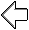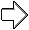previous page next page

special topics

## free computer programming text book project

Building a free downloadable text book on computer programming for university, college, community college, and high school classes in computer programming.

If you like the idea of this project,

send donations to:
Milo
PO Box 1361
Tustin, California 92781

Supporting the entire project:

If you have a business or organization that can support the entire cost of this project, please contact Pr Ntr Kmt (my church)

Some or all of the material on this web page appears in the

I do the news as an unpaid volunteer for KOCI 101.5 FM, Newport Beach/Costa Mesa (also available on the web)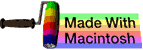This web site handcrafted on Macintosh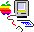computers using Tom Bender’s Tex-Edit Plus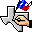and served using FreeBSD.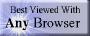Names and logos of various OSs are trademarks of their respective owners.